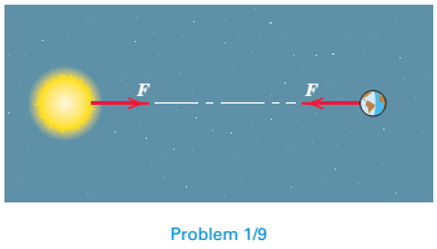#### Concept explainers#### Videos

Textbook QuestionChapter 1, Problem 9P

Compute the magnitude F of the force which the sun exerts on the earth. Perform the calculation first in pounds and then convert your result to newtons. Refer to Table D/2 in Appendix D for necessary physical quantities.05:16
Students have asked these similar questions
For the objects shown below sketch the object in the space provided after rotating it about the axis by the indicated amount.
the magnitudes of the three forces applied to the eye are T1 = 110 lb, T2 = 40 lg and T3 = 150 lb. Replace these forces with a single equivalent force R. Show result in a sketch of the eye
torque (or moment) is defined as a force acting perpendicular to a moment arm about a moment center and is defined as: Torque = Force x Distance. A technician is required to apply a torque of 360 lb.·in. (pound·inch) to a series of bolts in an assembly. As part of the technical report that needs to accompany the assembly, you must convert this value to lb.·ft. (pound·foot) and N·m. (Netwon·meter).

Find more solutions based on key concepts
Knowledge BoosterRecommended textbooks for you
•International Edition---engineering Mechanics: St...
Mechanical Engineering
ISBN:9781305501607
Author:Andrew Pytel And Jaan Kiusalaas
Publisher:CENGAGE L
•International Edition---engineering Mechanics: St...
Mechanical Engineering
ISBN:9781305501607
Author:Andrew Pytel And Jaan Kiusalaas
Publisher:CENGAGE L